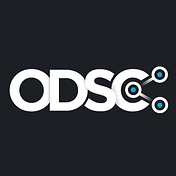# Data Science Applied to Wearables: Can Your Watch Help You Improve Your Lifestyle?

`token <- fitbitr::oauth_token(language = "en_US")`
`df_hr <- get_heart_rate_intraday_time_series_multiple(token = token, date_start = "2020-01-05", date_end = "2020-01-10")df_hr\$time <- times(df_hr\$time)kable(head(df_hr)) %>% kable_styling(position = "center", full_width = F)`
`breaks2 <- c(0.0000000, 0.2500000, 0.500000, 0.7500000, 0.9999999)labels2 <- times(breaks2)ggplot(df_hr, aes(x = time, y = value, col = as.factor(day))) +   geom_line(alpha = 0.7) +  scale_x_continuous(labels = labels2, breaks = breaks2) +  ylab("Heart rate") +  guides(color = guide_legend(title = "Date")) +  theme_bw()`
`rhr <- get_activity_time_series(token, "restingHeartRate", date = as.character(Sys.Date()), period = "max")rhr\$dateTime <- as.Date(rhr\$dateTime)rhr\$value <- as.numeric(rhr\$value)ggplot(rhr, aes(x = dateTime, y = value)) +  geom_line(col = "gray") +  stat_smooth(aes(x = dateTime, y = value), method = "lm", formula = y ~ poly(x, 21), se = FALSE) +  theme_bw() +   ylab("RHR") +   xlab("")`

--

--## ODSC - Open Data Science

Our passion is bringing thousands of the best and brightest data scientists together under one roof for an incredible learning and networking experience.